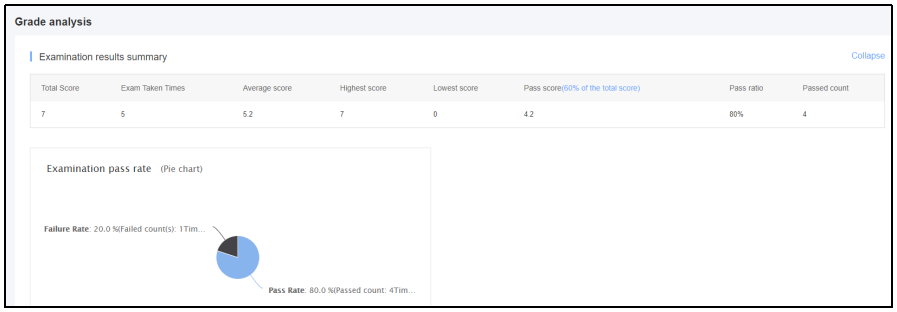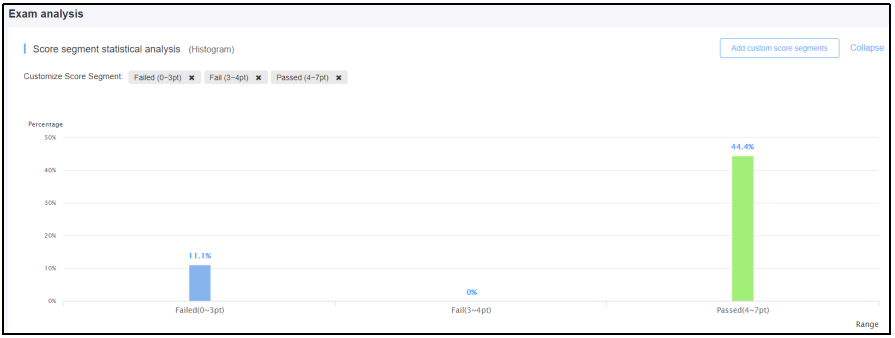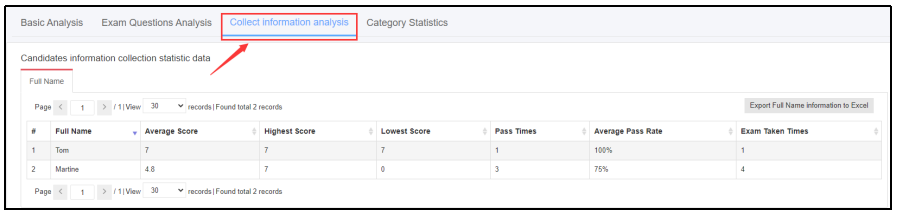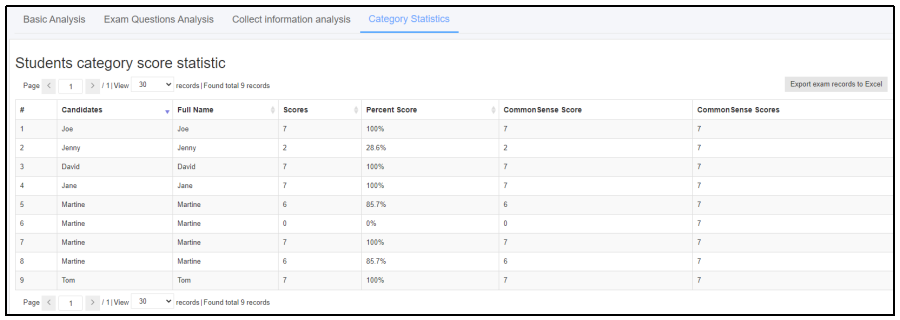## What Are the Advanteges of the Statistical Analysis Function of LAN Exam Maker?

Nowadays, much professional online examination software has powerful statistical analysis function. Therefore, after an online exam, statistical analysis will be offered by the online examination software. Let’s see what are the advantages of the powerful statistical analysis function of LAN Exam Maker.

Firstly, it supports rich statistical data and the chart analysis is clear. It includes basic analysis, exam questions analysis, analysis of collected candidates’ score and question category analysis.

There are two parts in basic analysis: grade analysis and exam analysis. Total score, exam taken times, average score, highest score, lowest score, pass rate and so on are provided in grade analysis by online examination software.In exam analysis, score segment statistical analysis, the correct answer rate in the category, the correct answer rate and the wrong answer rate of different question types are provided.Detailed question analysis of the exam are also provided by the online examination software. Users can check the category, times that the answer is answered incorrectly, exam taken times and the wrong answer rate of each questions of the exam.We can also view the statistics of each candidates, including the average score, highest score, lowest score, exam pass times, average pass rate and exam taken times.We can also view the score of each question category candidates get.Then with the powerful statistical analysis function of the LAN Exam Maker, teachers can be freed from the statistical analysis work after the exam,  which would really save much time and energy.

LAN Exam Maker is a professional and functional online examination software with powerful statistical analysis feature. And a free trial is now available; if you are interested, you can have a try!